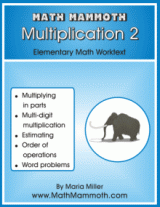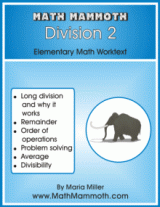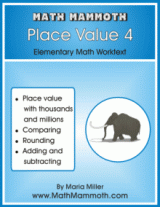### New Math Mammoth books: Multiplication 2, Division 2, Place Value 4

Some recent additions to Math Mammoth Blue Series books:## Math mammoth Multiplication 2

This book concentrates on multi-digit multiplication, first explaining what it is based on (multiplying in parts), then practicing the algorithm. also included: order of operations, multiplying with money, and lots of word problems.

See sample pages here: (PDF)

Contents & Introduction
Multiply by Whole Tens and Hundreds
Multiply in Parts
Multiplying in Columns, Standard Way
Error of Estimation
Order of Operations
Money and Change
Multiplying 3-digit by 2-digit## Math Mammoth Division 2

This book includes lessons on division, long division, the remainder, part problems, average, and problem solving.

See samples:
Contents and Introduction
Division Terms, Zero and One
Finding Parts with Division
Long Division 1
Long Division with 4-Digit Numbers
Average
Divisibility Rules

NOTE: Multiplication 2 and Division 2 now replace the earlier book called Multiplication Division 2.## Math Mammoth Place Value 4

In this book, the focus is on place value with
thousands, ten thousands, and hundred thousands. We also study numbers with millions a little. The book is most suitable for third or fourth grade.

See sample pages (PDF):
Contents
Thousands
Rounding
Estimating
A Little Bit of Millions